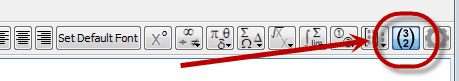﻿ Detect Column Vectors

# Detect Column Vectors

Top  Previous  Next

The FX Equation toolbar has one very important button.This button determines how FX Equation formats things that include commas.

When the button is on, FX Equation will format something like (4,3) as a column vector.  When it is off, FX Equation will leave (4,3) as an ordered pair.

This button controls an option (Detect Column Vectors) that used to be buried in the FX Equation options screen.  We have given it its own special button because it is something that you will often need to change.

Detecting column vectors also needs to be on for:

 • Matrices,
 • Piecewise Defined Functions,
 • Bounds of Definite Integrals

The Detect Column Vectors button has become less important with the introduction of Equation Contexts as this setting can now be managed using the context buttons.